# Ms. Harley Rides to School

Ms. Harley is a teacher who saves money by riding her motorcycle to school in the morning and back home in the afternoon. It costs Ms. Harley nine cents a mile to ride her motorcycle. Ms. Harley knows the distance from her house in Greenville to Lakeland is three and forty-five hundredths miles. The distance from Lakeland to Centerville is five and forty-eight hundredths miles. The distance from Centerville to Sunrise City is one and seven hundredths miles. The distance from Sunrise City to her school is one and five tenths miles. How many total miles does Ms. Harley ride to school and back home in one day? Ms. Harley rides to school and back home five days each week for four weeks. Ms. Harley has a gas budget of fifty dollars. Does Ms. Harley stay within her budget? Show all your mathematical thinking.

Assessment

## Plan

#### Formal Mathematical Language and Symbolic Notation

A student may independently select a printed number line, number chart, ten frames, graph paper, etc. as they work on a task.

### Suggested materials

Engagement Image:

Teachers may project the image below to launch this task for their students, define nouns, promote discussion, access prior knowledge, and inspire engagement and problem solving.

## Solutions tabs

### Expert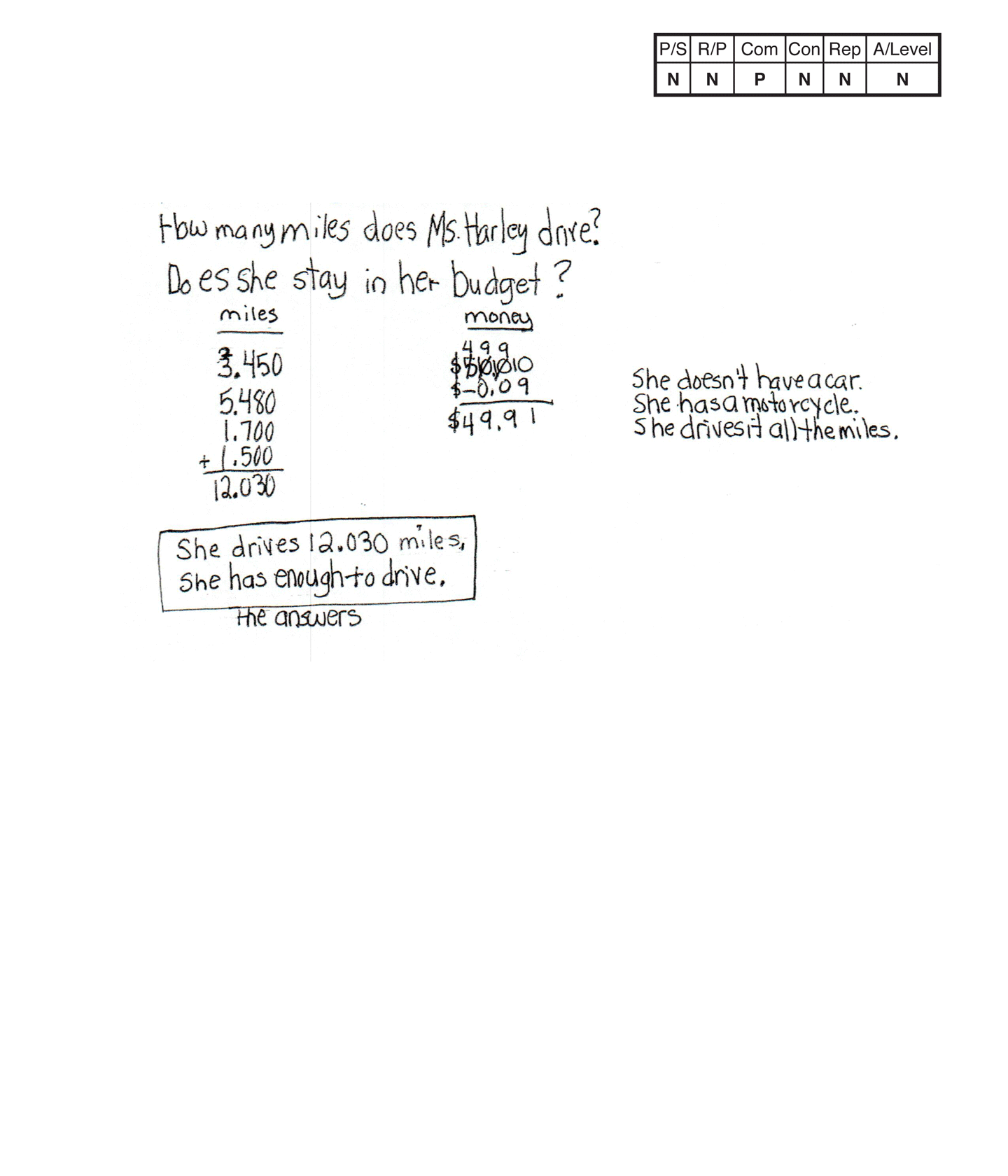This student does not meet the standard.

### Scoring Rationale

#### Novice

The student's strategy of listing the miles Ms. Harley rides to school in decimals to the thousandths and subtracting $0.09 from$50.00 does not work to solve the first and second parts of the task. The student's first answer, "She drives 12.030 miles," is not correct. The student's second answer, "She has enough to drive," is not correct as it is based on incorrect reasoning.

#### Novice

The student does not demonstrate correct reasoning and proof of the underlying concepts of the task. The student is not able to notate decimals to the hundreds and tenths place. It is not clear if the student's notation—1.500, represents one and five-tenths or one and five hundreds as the student lists one and seven hundredths as 1.700. The student does not understand that the miles Ms. Harley rides to school have to include total miles for a round trip. The student does not show correct reasoning that the total miles for 20 round trips has to be multiplied by $0.09 to determine if the cost does not exceed$50.00.

#### Practitioner

The student makes the mathematically relevant observation, "Lakeland to Centerville is most miles and costs the most gas."

#### Apprentice

The student's table is appropriate to the task but is not accurate. The student omits a decimal point for 1.50 miles. The student also omits a dollar sign for $0.14 and$0.80.

#### Apprentice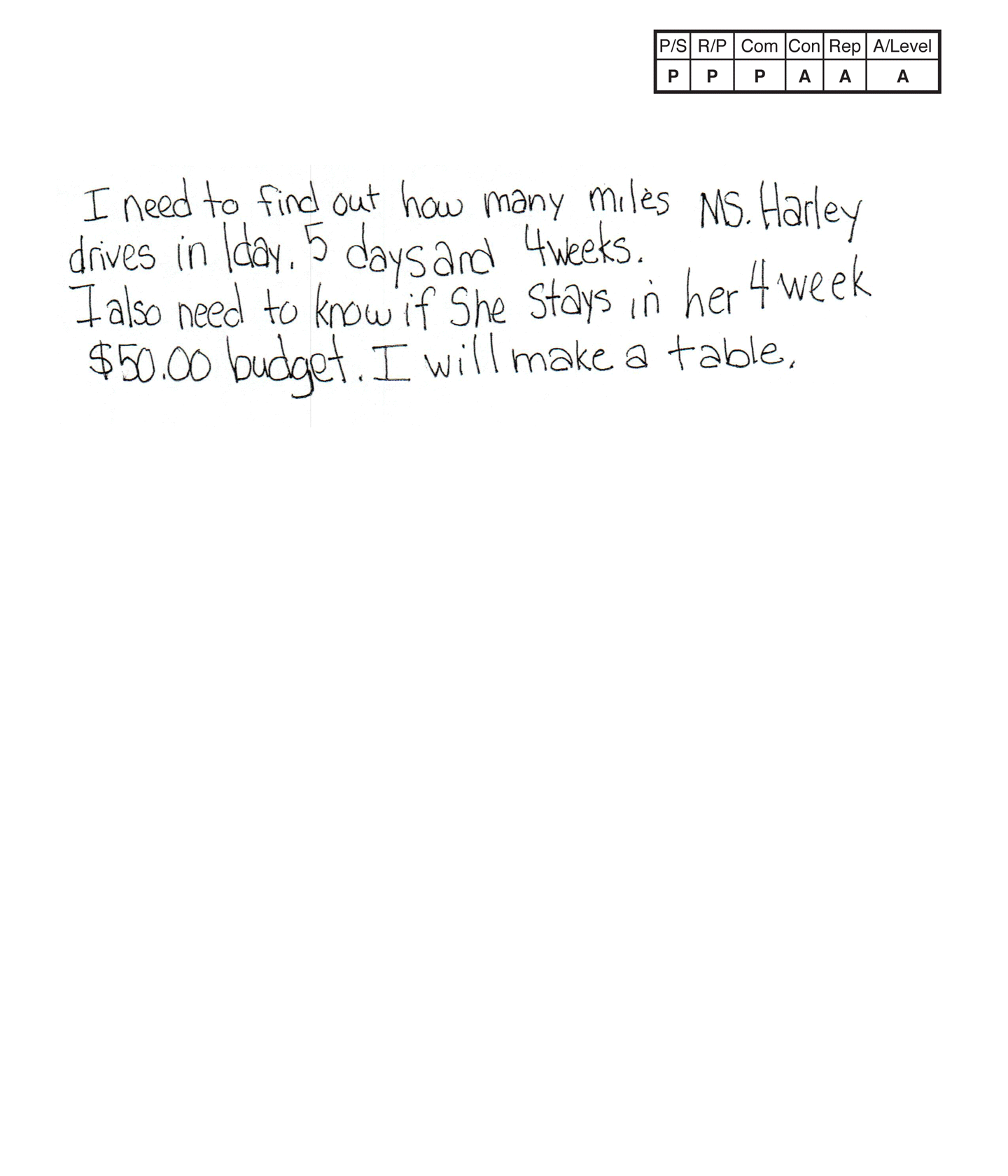,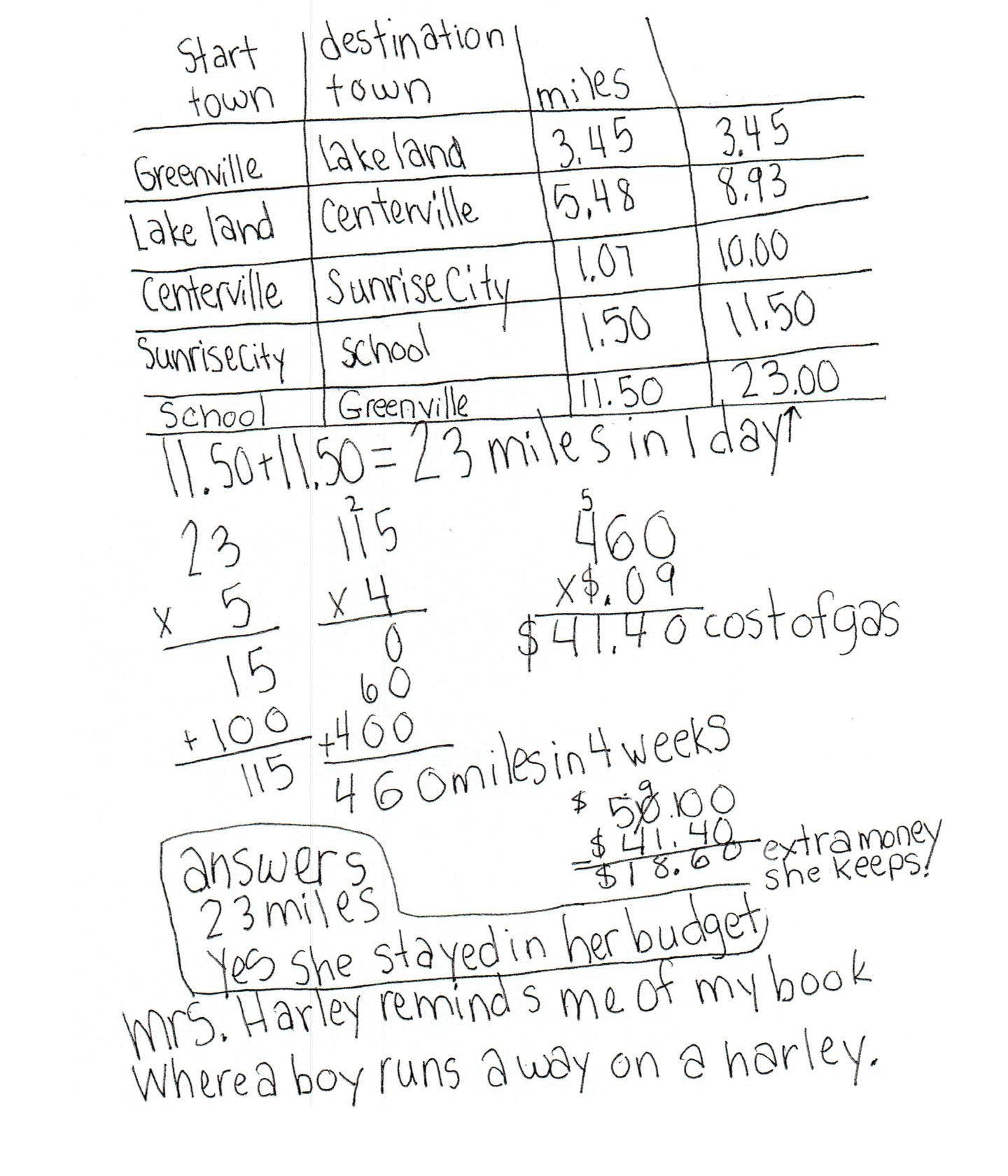This student does not meet the standard.

### Scoring Rationale

#### Practitioner

The student correctly uses the mathematical terms miles, days, weeks, cost from the task. The student also correctly uses the term table. The student correctly uses the mathematical notation 3.45, 5.48, 1.07, 1.50, 11.50, 8.93, 10.00, 23.00, $.09,$41.40, $50.00,$18.60.

#### Apprentice

The student attempts to find the difference between $50.00 and the cost of the 20 round trips,$41.40, but the student miscalculates and arrives at an incorrect connection. The student's statement, "Mrs. Harley reminds me of my book where a boy runs away on a harley," is not considered a mathematically relevant connection.

#### Apprentice

The student's table is appropriate to the task but is not accurate. The student's fourth column should be labeled "total miles," "running total of miles," or "total miles rode."

#### Apprentice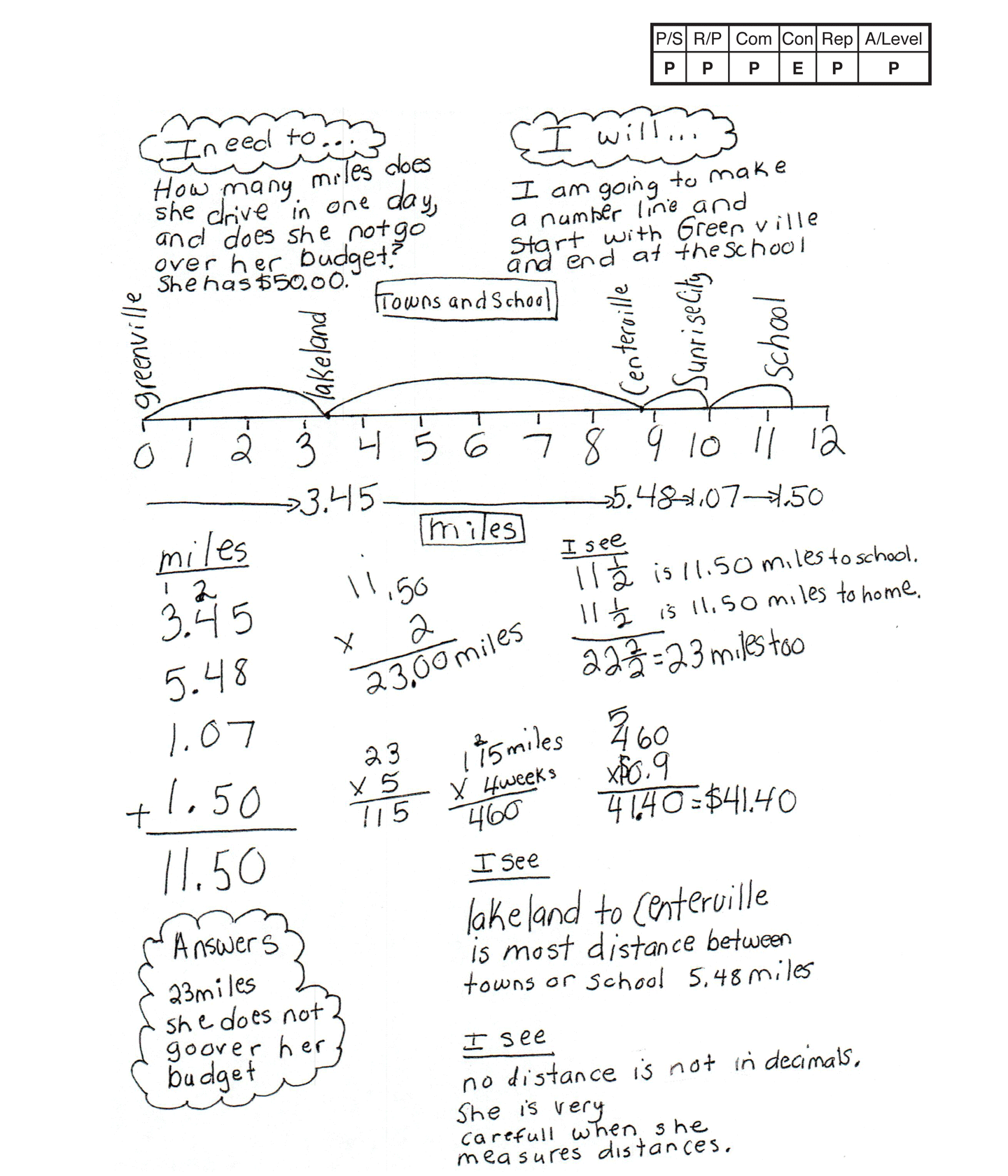This student meets the standard.

### Scoring Rationale

#### Practitioner

The student's strategy of using a number line to show the distance in miles Ms. Harley rides between locations from home to school works to solve the first part of the task. The student solves the rest of the task by computing the number of miles she drives in a round trip, in five days, four weeks and, how much money Ms. Harley uses for gas. The student's answers, "23 miles," and "she does not go over her budget," are correct.

#### Expert

The student makes mathematically relevant Practitioner connections. The student states, "Lakeland to Centerville is most distance between towns or school 5.48 miles," and "no distance is not in decimals. She is very careful when she measures distances." The student makes an Expert connection by using calculation to explain how fractions can replace decimals and arrive at the same answer of 23 miles. The student states "I see 11 1/2 is 11.50 miles to school + 11 1/2 is 11.50 miles to home = 22 2/2 = 23 miles too."

#### Practitioner

The student's number line is appropriate to the task and accurate. All labels are included, the intervals are accurate, and the "jumps" are correct.

#### Practitioner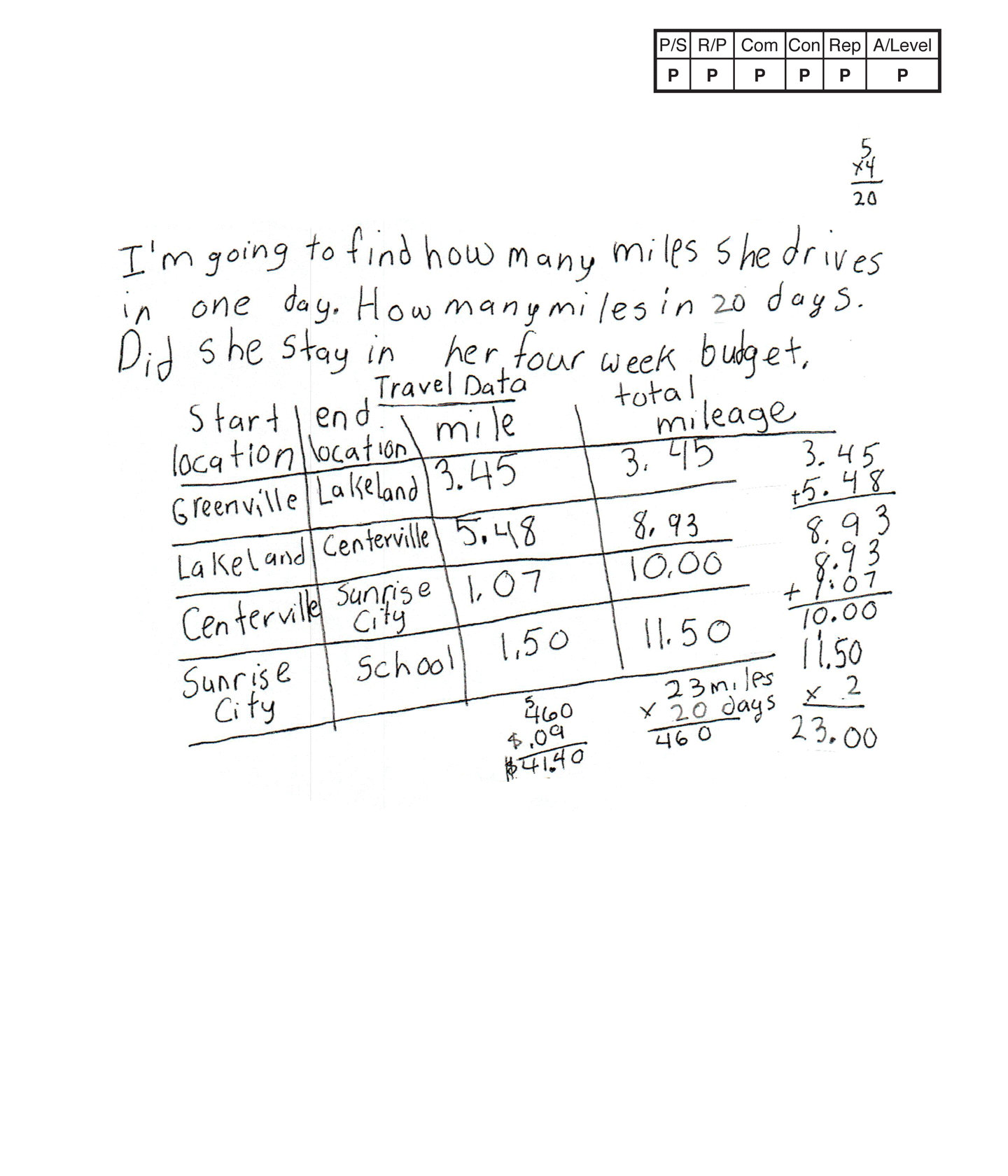,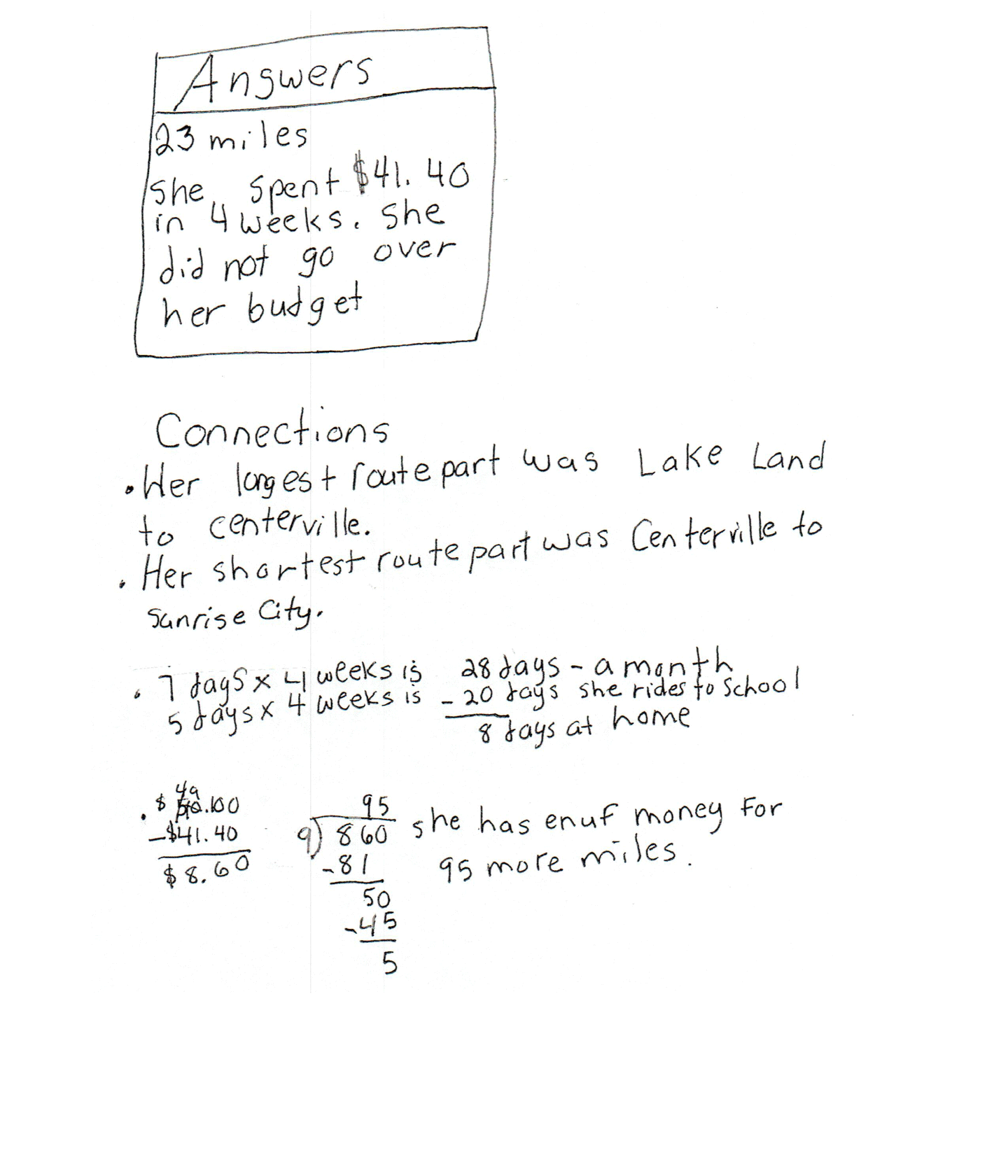This student meets the standard.

### Scoring Rationale

#### Practitioner

The student's table is appropriate to the task and accurate. All labels are included and all entered data is correct.

#### Practitioner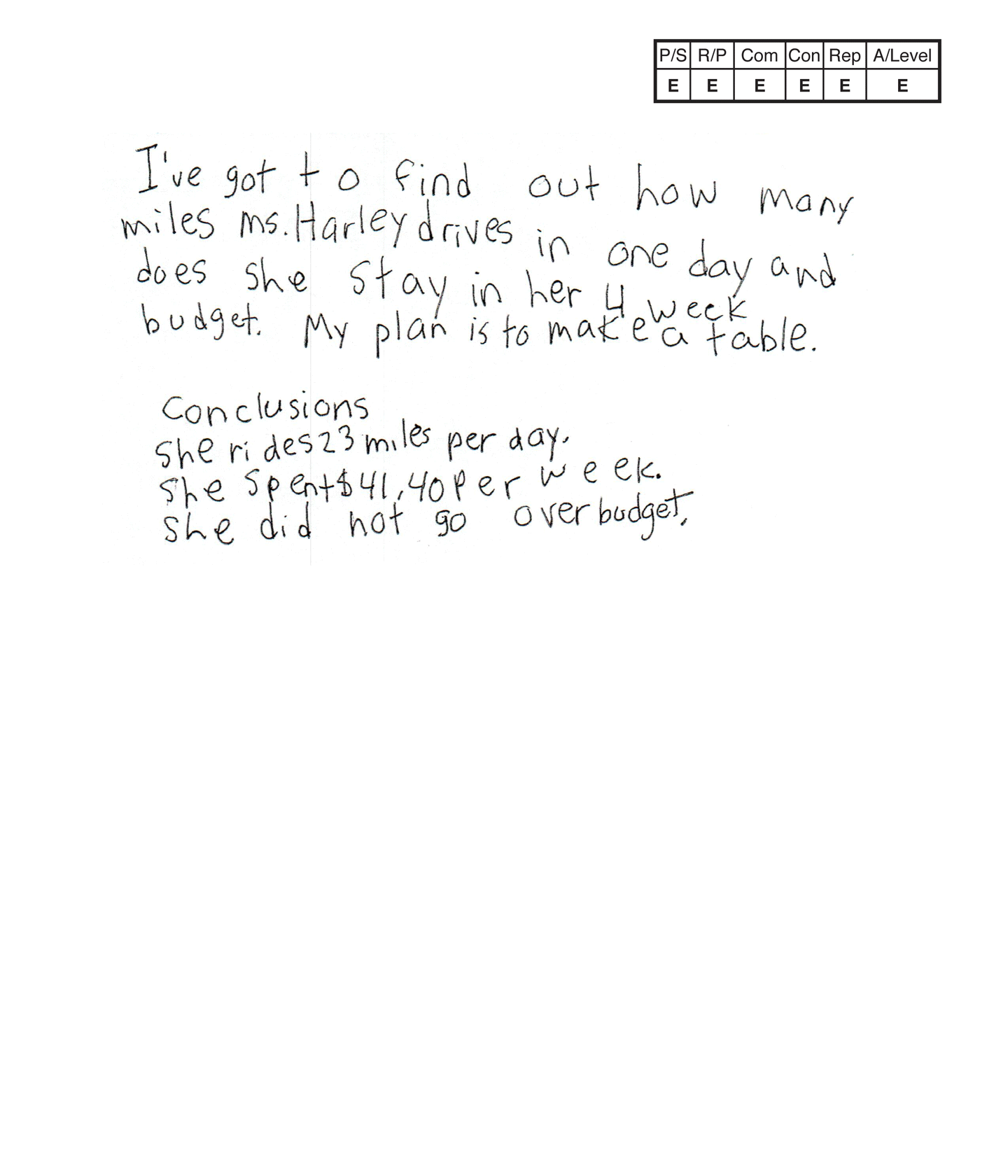,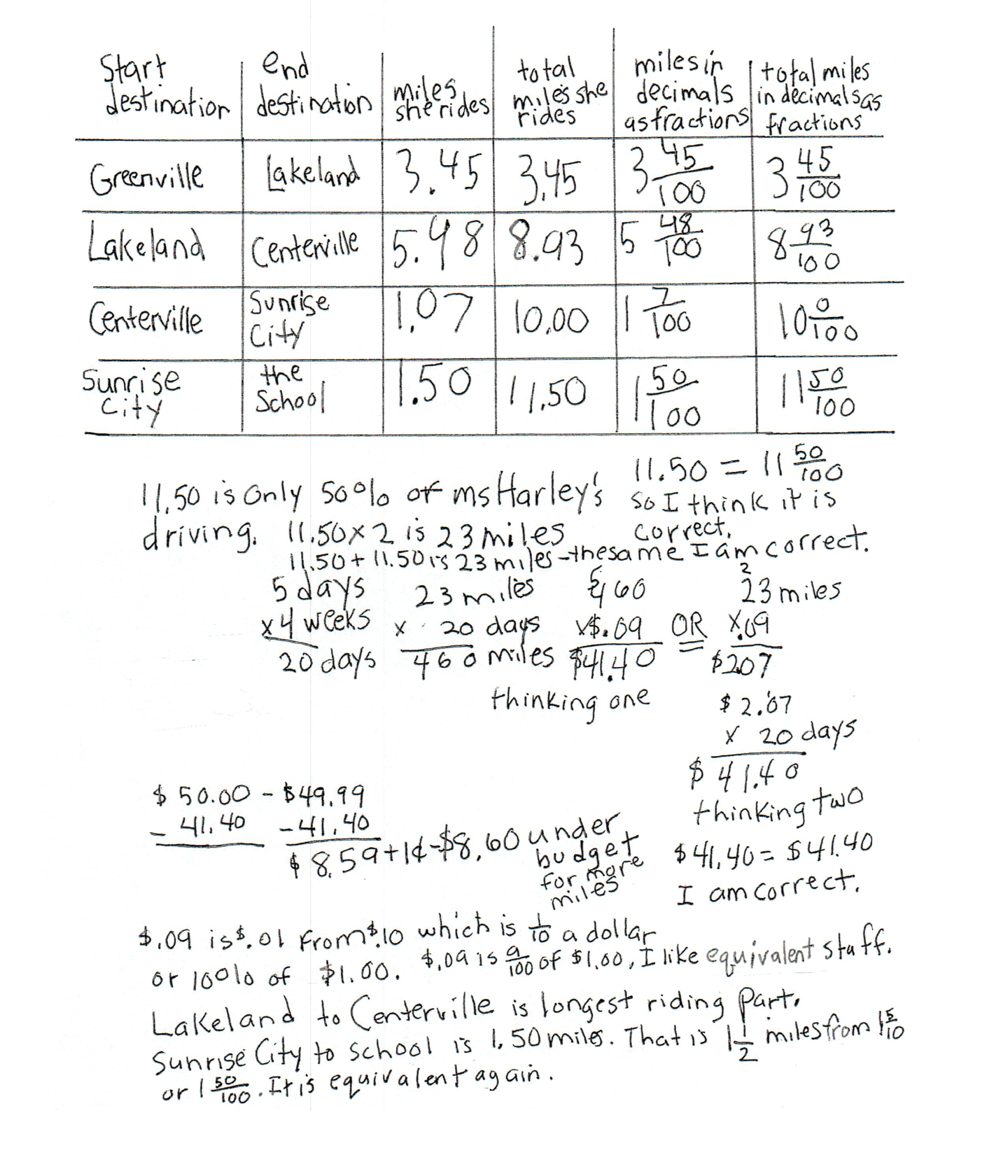This student exceeds the standard.

### Scoring Rationale

#### Expert

The student makes mathematically relevant Practitioner connections. The student states, "Lakeland to Centerville is longest riding part," and "$8.60 under budget for more miles." The student makes Expert connections. The student includes the equivalent mixed numbers for "miles in decimals as fractions" and "total miles in decimals as fractions" on their table. The student justifies their decisions. The student states, "11.50 if only 50% of ms Harley's driving. 11.50 x 2 is 23 miles," "11.50 + 11.50 is 23 miles—the same, I am correct," "11.50 = 11 50/100 so I think it is correct." The students uses two different strategies for computing$41.40 calling them, "thinking one" and "thinking two" and stating, "$41.40 =$41.40 I am correct." The student also supports their understanding that, "$.09 is$.01 from $.10 which is 1/10 of a dollar or 10% of$1.00," and "$.09 is 9/100 of$1.00. I like equivalent stuff." The student ends their solution by stating, "Sunrise City to school is 1.50 miles. That is 1 1/2 miles from 1 5/10 or 1 50/100. It is equivalent again."

#### Expert

The student's table is appropriate to the task and accurate. All labels are included and all extended data is correct. The student extends their thinking to include mixed numbers in the table and to compare 11.50 from the table to 50% and 1.50 from the table to 1 1/2 and 1 5/10 in their solution.

#### Expert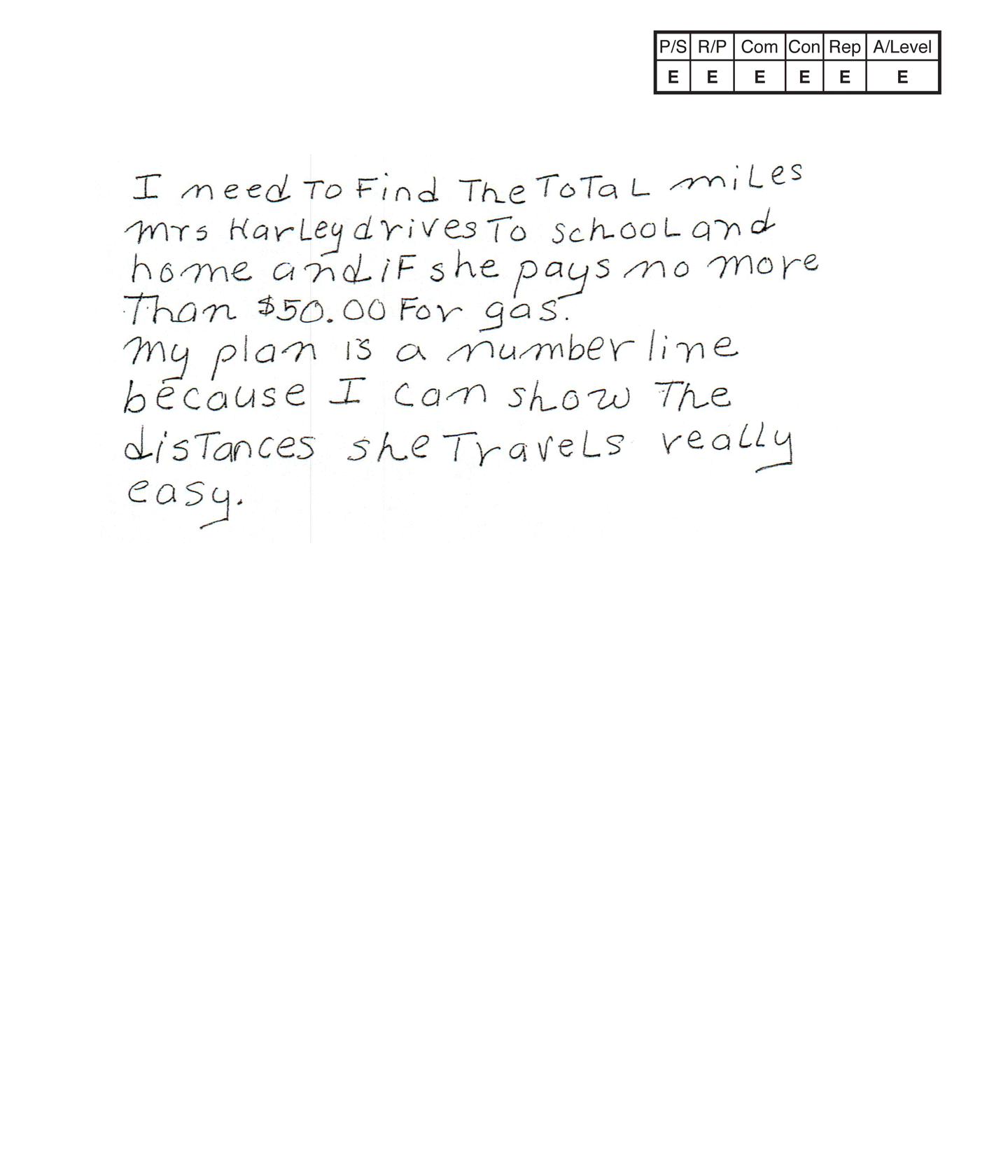,,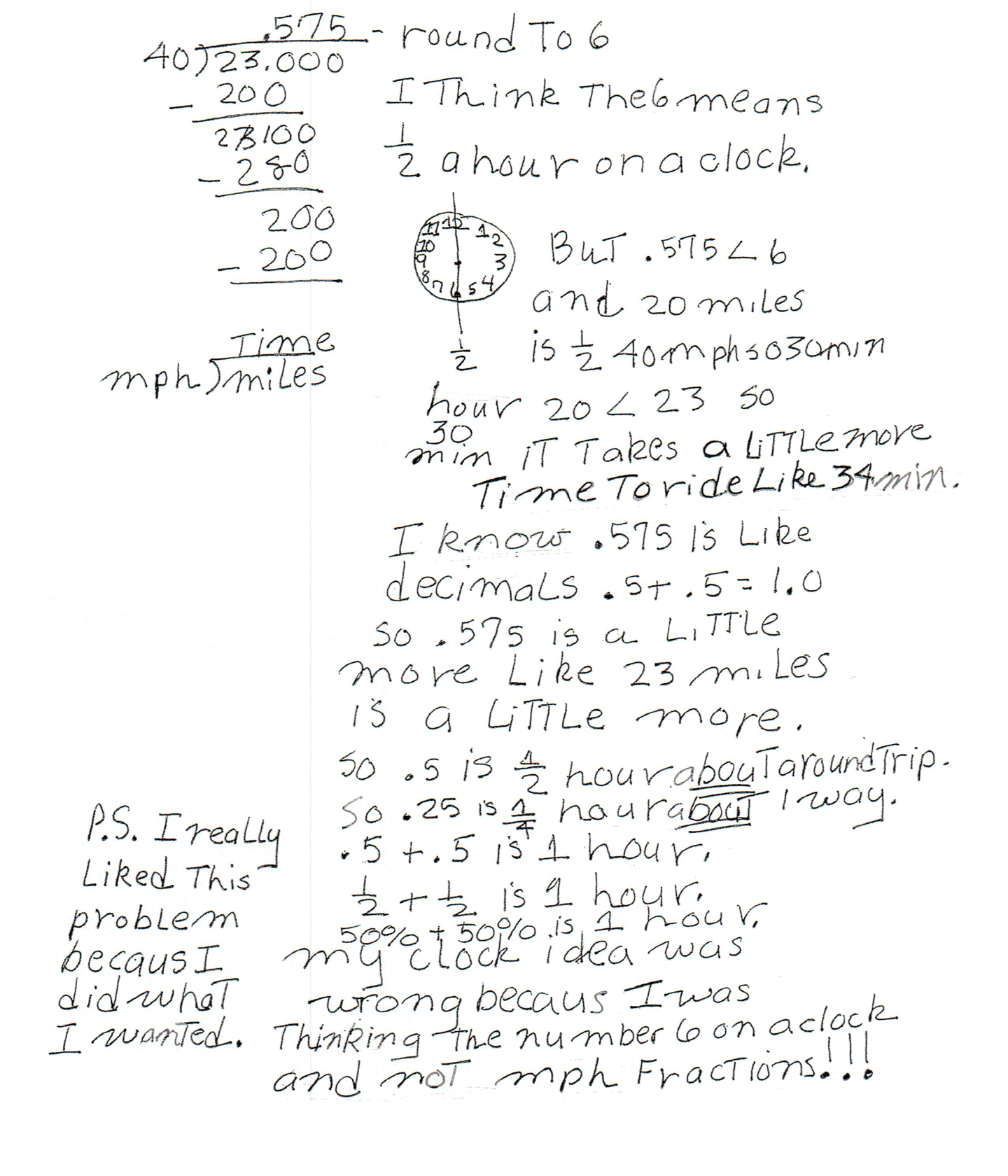This student exceeds the standard.

### Scoring Rationale

#### Expert

The student's strategy of using a number line and calculation to show the total distance in miles Ms. Harley rides between locations from home to school and home, works to solve the first part of the task. The student's strategy of calculating the cost of gas to ride 23 miles and the cost for riding 20 days, works to solve the second part of the task.The student's answers, "3 miles," and "Mrs Harley does not go over her budget," are correct. The student continues their solution to include percents and time measurement.

#### Expert

The student demonstrates correct reasoning of the underlying concepts of the task. The student correctly uses decimal notation to define the four distances in hundredths and tenths of a mile, and the total miles Ms. Harley travels to school and home in one day and 20 days. The student demonstrates correct reasoning by computing and using money notation to determine $41.40 as the total cost of gas needed for 20 days and comparing that total to$50.00. The student also demonstrates understanding of the concepts of fractions, percents and time measurement. The student justifies their understanding of comparing time to miles per hour and decimals and reaches a conclusion.

#### Expert

The student correctly uses the mathematical terms miles, distance, total, days, weeks, cost from the task. The student also correctly uses the terms number line time, per, miles per hour/mph, time, hour, min., more, decimals, fraction. The student correctly uses the mathematical notation 3.45, 5.48, 1.07, 1.50, 11.50, 23.00, .575, .5, 1.0, $.09,$2.07, $41.40,$50.00, 1/2, 1/4, 50%, 25%. <.

#### Expert

The student solves the task and makes the Expert connection of extending their thinking to miles per hour and how this concept relates to the task. The student states, "If she drives 40 mph you can find the time she rides." The student defines 40 mph and determines "20 miles is 1/2 hour or 50% of a hour or 30 min.," "10 miles is 1/4 hour or 25% of a hour or 15 min.," "So 11.50 miles is about 15 min. (1 way) and 23 miles is about 30 min. (round trip)." The student divides 23.00 miles by 40 miles per hour for a quotient of .575 which the student rounds to 6. The student states, "I think the 6 means 1/2 hour on a clock," The student diagrams a clock and states, "But .545 < 6 and 20 miles is 1/2 40 mph so 30 mins, 20 < 23 so it takes a little more time to ride like 34 min." The student continues their explanation. "I know .575 is like decimals. .5 + .5 = 1.0 so .575 is a little more like 23 miles is a little more. So .5 is 1/2 hour about a round trip. So .25 is 1/4 hour about 1 way." The student aligns decimals and fractions to percents. ".5 + .5 is 1 hour. 1/2 + 1/2 is 1 hour. 50% + 50% is 1 hour." The student concludes, "my clock idea was wrong becaus I was thinking the number 6 on a clock and not mph fractions!!!"

#### Expert

The student's table is appropriate to the task and accurate. All labels are included and all entered data is correct. The student extends their thinking to include mixed numbers in the table and to compare 11.50 from the table to 50% of 23.00, and 1.50 from the table to 1 1/2 and 1 5/10 in their solution. The student also diagrams a clock to indicate 1/2 an hour representing 30 minutes.